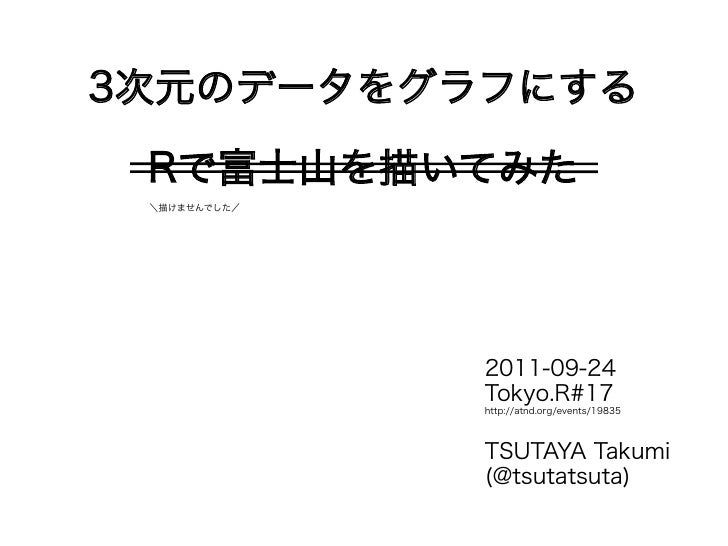Successfully reported this slideshow.Upcoming SlideShare
×

# 3次元のデータをグラフにする(Tokyo.R#17)

13,626 views

Published on

Rを使って3次元のデータをグラフにする方法を簡単にまとめました．

Published in: Education
• Full Name
Comment goes here.

Are you sure you want to Yes No• Be the first to comment

### 3次元のデータをグラフにする(Tokyo.R#17)

1. 1. 3次元のデータをグラフにする Rで富士山を描いてみた ＼描けませんでした／ 2011-09-24 Tokyo.R#17 http://atnd.org/events/19835 TSUTAYA Takumi (@tsutatsuta)
2. 2. 自己紹介蔦谷 匠TSUTAYA Takumi・自然人類学の研究など・大学院生のような http://tsutatsuta.web.fc2.com/
3. 3. 発表の内容● 何を図示するときに使うか● 3次元のデータをグラフにする・ 今回使用するデータセット・ contour・ image・ persp・ scatterplot3d● まとめ
4. 4. 何を図示するときに使うか ● 2つの変数をもつ関数 ● 2つ以上の変数をもつ関数 ● 座標と標高 …などなど 厳密な比較には向かないが イメージをつかみやすいたしかに三次元図は見栄えが良く、論文の飾りに好適だが、しばしばそれだけに留まるので注意がいるか。人間の視覚は二次元対象で最も鋭敏のようである。 - グラフィックス参考実例集：三次元散布図 (RjpWiki)
5. 5. 今回使用するデータセット● volcano・ Maugna Whau 山 (Auckland) の標高データ・ 10m × 10m グリッド (Max: 195, Min: 94)・ 87行 (南→北) 61列 (東→西) Google Earth
6. 6. # データの準備# データのラベルを作成south.north <- 1:nrow(volcano) * 10 # rowの数は87east.west <- 1:ncol(volcano) * 10 # columnの数は61# 頂上の位置を変数に入れておくmt.top <- which(volcano == max(volcano), arr.ind = TRUE) * 10# 標高150mラインlevel150 <- which(volcano == 150, arr.ind = TRUE) * 10# scatterplot3dパッケージの読み込みlibrary(scatterplot3d)# http://cran.r-project.org/web/packages/scatterplot3d/index.html# scatterplot3d用ラベルの準備south.north.3d <- rep(south.north, length(east.west))west.east.3d <- vector(length = 87 * 61)for(i in 1:61){ west.east.3d[((i - 1) * 87 + 1):(i * 87)] <- east.west[i]}
7. 7. contour image
8. 8. contour● 等高線を描く・ 線の数や間隔を調整できる・ 線に数字を示すこともできる > ?contour より
9. 9. image● 値に応じた色を格子上に示す・ 色調や濃さを調整できる・ contour と相性が良い > ?image より
10. 10. #1a #1b#1c #1d
11. 11. # 1a # 1bimage(south.north, east.west, volcano, image(south.north, east.west, volcano, col = terrain.colors(100), col = rainbow(100), xlab = "South-North", ylab = "East-West") xlab = "South-North", ylab = "East-West")contour(south.north, east.west, volcano, contour(south.north, east.west, volcano, levels = c(175, 145), add = TRUE) levels = 160, lty = "dotted", add = TRUE)points(mt.top, mt.top, pch = 20, lines(c(0, 1000), c(150, 500)) col = "blue") # 頂上を図示 # (0, 150)と(1000, 150)を通る直線を引く# 1c # 1dimage(south.north, east.west, volcano, image(south.north, east.west, volcano, col = gray((10:0)/10), col = gray((0:100)/100), xlab = "South-North", ylab = "East-West") xlab = "South-North", ylab = "East-West")contour(south.north, east.west, volcano, contour(south.north, east.west, volcano, levels = 175, col = "red", add = TRUE) col = rainbow(10), add = TRUE)points(level150[ , 1], level150[ , 2], pch = 20, text(mt.top, mt.top, "TOP", col = "blue") col = "blue") # 標高150mの点を図示 # 頂上に"TOP"をプロット
12. 12. persp
13. 13. persp● 投影図を描く・ 立体的な図・ 陰をつけたりもできる > ?persp より
14. 14. #2a #2b#2c #2d
15. 15. #2a #2bmt.mw <- persp(south.north, east.west, mt.mw <- persp(south.north, east.west, volcano, theta = 25, phi = 30, scale = FALSE, volcano, theta = -25, phi = 50, scale = FALSE, col = "green", border = NA, ltheta = 120, col = "green", border = NA, ltheta = 120, shade = 0.7, ticktype = "detailed", shade = 0.5, ticktype = "detailed", cex.axis = 0.8, xlab = "South-North", cex.axis = 0.8, xlab = "South-North", ylab = "East-West", zlab = "Altitude") ylab = "East-West", zlab = "Altitude")points(trans3d(mt.top, mt.top, max(volcano), x <- 6pmat = mt.mw), col = "red", pch = 16) lines(trans3d(c(mt.top, level150[x, 1]), # 頂上を図示 c(mt.top, level150[x, 2]), c(max(volcano), 150), pmat = mt.mw), col = "blue") # 頂上と，標高150mにある一点を結ぶ#2c #2dmt.mw <- persp(south.north, east.west, mt.mw <- persp(south.north, east.west, volcano, theta = 25, phi = 30, scale = FALSE, volcano, theta = 25, phi = 30, scale = FALSE, col = NA, border = "black", ltheta = 120, col = rainbow(7), border = NA, ltheta = -120, shade = 0.3, ticktype = "detailed", shade = 0.7, ticktype = "detailed", cex.axis = 0.8, xlab = "South-North", cex.axis = 0.8, xlab = "South-North", ylab = "East-West", zlab = "Altitude") ylab = "East-West", zlab = "Altitude")x <- 6 x <- 6points(trans3d(level150[ , 1], level150[ , 2], 150, text(trans3d(mt.top, mt.top, max(volcano), pmat = mt.mw), col = "red", pch = 16) pmat = mt.mw), "TOP", pch = 16) # 標高150mの点を図示 # 頂上に"TOP"をプロット
16. 16. scatterplot3d
17. 17. scatterplot3d● 3次元空間へのプロット・ scatterplot3dパッケージが必要・ http://cran.r-project.org/web/packages/scatterplot3d/index.html > ?scatterplot3dより
18. 18. #3a #3b#3c #3d
19. 19. # 3a # 3bmt.mw.3d <- scatterplot3d(west.east.3d, level145to150.3d <- which(volcano < 150 & south.north.3d, volcano, scale.y = 1, volcano >= 145) # 標高145m以上150m未満 highlight.3d = TRUE, level150to155.3d <- which(volcano < 155 & zlim = c(80, 200), xlab = "West-East", volcano >= 150) # 標高150m以上145m未満 ylab = "South-North", zlab = "Altitude") mt.mw.3d<- scatterplot3d(mt.mw.3d\$plane3d(150, x.coef = 0, y.coef = 0, west.east.3d[level145to150.3d], lty.box = "dashed") south.north.3d[level145to150.3d], # 標高150mラインに平面の追加． volcano[level145to150.3d], color = "red",mt.mw.3d\$points3d(mt.top, mt.top, scale.y = 1, xlim = c(0, 700), ylim = c(0, 1000), max(volcano), col = "blue", pch = 19) zlim = c(80, 200), xlab = "West-East", # 頂上の追加． ylab = "South-North", zlab = "Altitude") mt.mw.3d\$plane3d(150, x.coef = 0, y.coef = 0, lty.box = "dashed") # 標高150mラインに平面 mt.mw.3d\$points3d(west.east.3d[level150to155.3d], south.north.3d[level150to155.3d], volcano[level150to155.3d], col = "red") # 標高150m以上155m未満# 3cwest275to300.3d <- which(west.east.3d < 300 & west.east.3d >= 275) # 西から275以上300m未満west500to525.3d <- which(west.east.3d < 525 & # 3d west.east.3d >= 500) # 西から500以上525m未満 south275to325.3d <- which(south.north.3d < 325 &label.3d <- c(west275to300.3d, west500to525.3d) south.north.3d >= 275) # 南から275以上325m未満mt.mw.3d <- scatterplot3d(west.east.3d[label.3d], mt.mw.3d <-scatterplot3d( south.north.3d[label.3d], volcano[label.3d], west.east.3d[south275to325.3d], scale.y = 1.5, highlight.3d = TRUE, south.north.3d[south275to325.3d], xlim = c(0, 700), ylim = c(0, 1000), volcano[south275to325.3d], scale.y = 0.7, zlim = c(80, 200), xlab = "West-East", color = "blue", xlim = c(0, 700), ylim = c(0, 1000), ylab = "South-North", zlab = "Altitude") zlim = c(80, 200), xlab = "West-East", ylab = "South-North", zlab = "Altitude")
20. 20. まとめ 図示の形式 定量性 イメージcontourimage 連続的な面 ◯ △persp 連続的な面 △ ◯scatterplot3d 離散的な点 △ ◯使わないで済むなら、それに越したことはないかもしれません…
21. 21. 参考● Rヘルプドキュメント● 高水準作図関数 (R-Tips) http://cse.naro.affrc.go.jp/takezawa/r-tips/r/50.html● 三次元散布図 (RjpWiki) http://www.okada.jp.org/RWiki/他にも関数やパッケージをご存じでしたら、ぜひ教えてください！